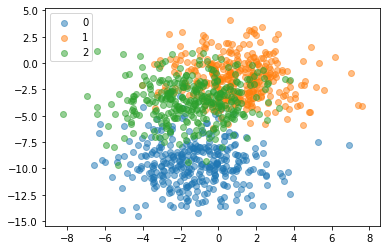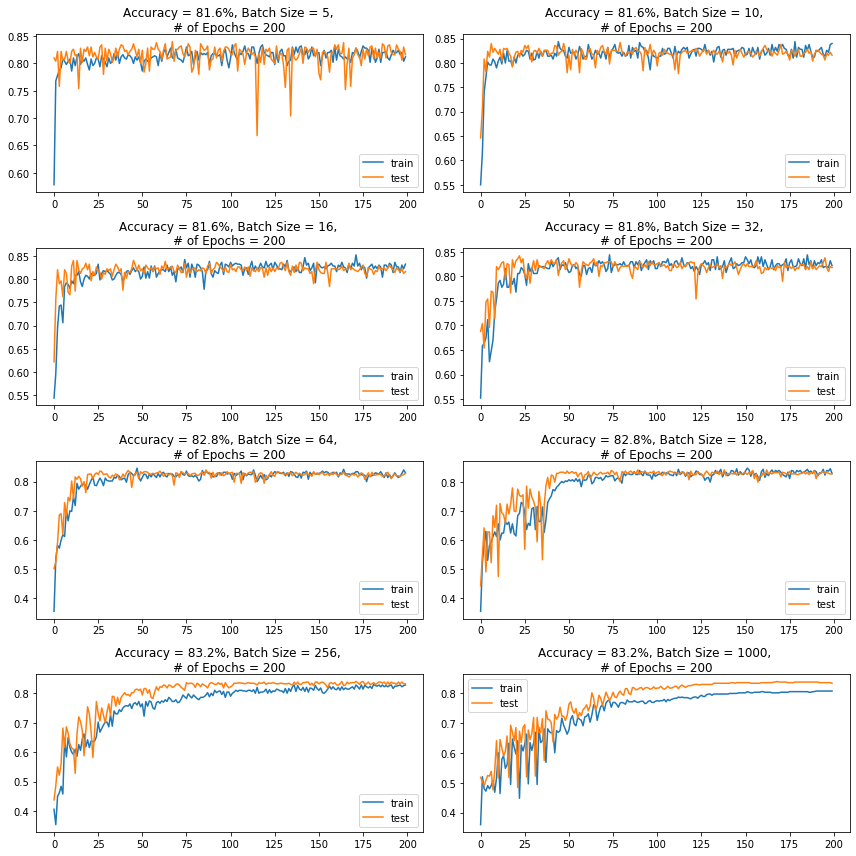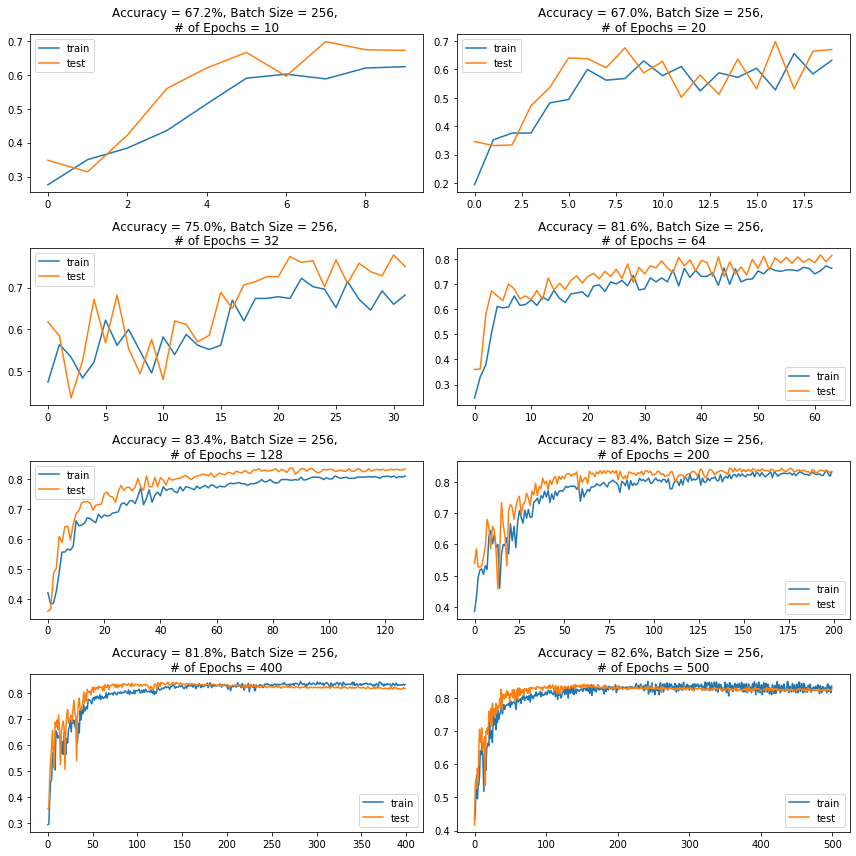# Batch Size, Epochs and Their Effect on Learning¶

## What Is a Batch?¶

Neural networks are trained using gradient descent where the estimate of the error is used to update the weights and is calculated based on a subset of the training dataset. The number of examples from the training dataset used in the estimate of the error gradient is called the batch size and is an important hyperparameter that influences the dynamics of the learning algorithm.

• Batch size controls the accuracy of the estimate of the error gradient when training neural networks.
• There is a tension between batch size and the speed and stability of the learning process.

### Flavors of Batch Gradient Descent:¶

• Batch Gradient Descent: Batch Size = Size of Training Set
• Stochastic Gradient Descent: Batch Size = $1$
• Mini-Batch Gradient Descent: Batch size is set to more than one and less than the total number of examples in the training dataset

In the case of mini-batch gradient descent, popular batch sizes include $32$, $64$, and $128$ samples. You may see these values used in models often in deep learning literature.

• The frequent updates immediately give an insight into the performance of the model and the rate of improvement.
• The increased model update frequency can result in faster learning on some problems.
• The noisy update process can allow the model to avoid local minima (e.g. premature convergence).

Downsides:

• Updating the model so frequently is more computationally expensive than other configurations of gradient descent, taking significantly longer to train models on large datasets.
• The frequent updates can result in a noisy gradient signal, which may cause the model parameters and in turn the model error to jump around (have a higher variance over training epochs).
• The noisy learning process down the error gradient can also make it hard for the algorithm to settle on an error minimum for the model.

• Fewer updates to the model means this variant of gradient descent is more computationally efficient than stochastic gradient descent.
• The decreased update frequency results in a more stable error gradient and may result in a more stable convergence on some problems.
• The separation of the calculation of prediction errors and the model update lends the algorithm to parallel processing based implementations.

• The more stable error gradient may result in premature convergence of the model to a less optimal set of parameters.
• The updates at the end of the training epoch require the additional complexity of accumulating prediction errors across all training examples.
• Commonly, batch gradient descent is implemented in such a way that it requires the entire training dataset in memory and available to the algorithm.
• Model updates, and in turn training speed, may become very slow for large datasets.

• The model update frequency is higher than batch gradient descent which allows for a more robust convergence, avoiding local minima.
• The batched updates provide a computationally more efficient process than stochastic gradient descent.
• The batching allows both the efficiency of not having all training data in memory and algorithm implementations.

• Mini-batch requires the configuration of an additional “mini-batch size” hyperparameter for the learning algorithm.
• Error information must be accumulated across mini-batches of training examples like batch gradient descent.

## Optimizing Batch Size Multi-Class Classification Problem¶

We will use a small multi-class classification problem as the basis to demonstrate the effect of batch size on learning.

The scikit-learn class provides the make_blobs() function that can be used to create a multi-class classification problem with the prescribed number of samples, input variables, classes, and variance of samples within a class.

The problem can be configured to have two input variables (to represent the $x$ and $y$ coordinates of the points) and a standard deviation of $2.0$ for points within each group. We will use the same random state (seed for the pseudorandom number generator) to ensure that we always get the same data points.

In :
# scatter plot of blobs dataset
from sklearn.datasets import make_blobs
import matplotlib.pyplot as plt
import numpy as np

# generate 2d classification dataset
X, y = make_blobs(n_samples=1000, centers=3, n_features=2, cluster_std=2, random_state=2)

# scatter plot for each class value
for class_value in range(3):

# select indices of points with the class label
row_ix = np.where(y == class_value)

# scatter plot for points with a different color
plt.scatter(X[row_ix, 0], X[row_ix, 1], label=class_value, alpha=0.5)
plt.legend()

# show plot
plt.show()We can see that the standard deviation of $2.0$ means that the classes are not linearly separable (separable by a line) causing many ambiguous points.

This is desirable as it means that the problem is non-trivial and will allow a neural network model to find many different “good enough” candidate solutions.

In :
from keras.layers import Dense
from keras.models import Sequential
from keras.optimizers import SGD
from keras.utils import to_categorical

# prepare train and test dataset
def prepare_data():

# generate 2d classification dataset
X, y = make_blobs(n_samples=1000, centers=3, n_features=2, cluster_std=2, random_state=2)

# one hot encode output variable
y = to_categorical(y)

# split into train and test
n_train = 500
train_X, test_X = X[:n_train, :], X[n_train:, :]
train_y, test_y = y[:n_train], y[n_train:]
return train_X, train_y, test_X, test_y

# fit a model and plot learning curve
def fit_model(train_X, train_y, test_X, test_y, n_batch, epochs, opt, opt_name='SGD'):

# define model
model = Sequential()

# compile model
model.compile(loss='categorical_crossentropy', optimizer=opt, metrics=['accuracy'])

# fit model
history = model.fit(train_X, train_y, validation_data=(test_X, test_y), epochs=epochs, verbose=0,
batch_size=n_batch)

# evaluate the model
scores = model.evaluate(test_X, test_y)
accuracy = round(scores * 100, 3)

# plot learning curves
plt.plot(history.history['accuracy'], label='train')
plt.plot(history.history['val_accuracy'], label='test')
plt.title(f'Accuracy = {accuracy}%, Batch Size = {n_batch}, \n# of Epochs = {epochs}',

plt.legend()

Using TensorFlow backend.

In :
# prepare dataset
train_X, train_y, test_X, test_y = prepare_data()

# create learning curves for different batch sizes
batch_sizes = [5, 10, 16, 32, 64, 128, 256, len(X)]

# set optimizer
opt = SGD(lr=0.01, momentum=0.9)

plt.figure(figsize=(12,12))

for i in range(len(batch_sizes)):

# determine the plot number
plot_no = 420 + (i+1)
plt.subplot(plot_no)

# fit model and plot learning curves for a batch size
fit_model(train_X, train_y, test_X, test_y, batch_sizes[i], epochs=200, opt=opt)

# show learning curves
plt.tight_layout()
plt.show()

500/500 [==============================] - 0s 12us/step
500/500 [==============================] - 0s 12us/step
500/500 [==============================] - 0s 12us/step
500/500 [==============================] - 0s 11us/step
500/500 [==============================] - 0s 11us/step
500/500 [==============================] - 0s 11us/step
500/500 [==============================] - 0s 12us/step
500/500 [==============================] - 0s 10us/stepRunning the example creates a figure with eight line plots showing the classification accuracy on the train and test sets of models with different batch sizes when using mini-batch gradient descent.

The plots show that small batch results generally in rapid learning but a volatile learning process with higher variance in the classification accuracy. Larger batch sizes slow down the learning process but the final stages result in a convergence to a more stable model exemplified by lower variance in classification accuracy.

## What Is an Epoch?¶

The number of epochs is a hyperparameter that defines the number times that the learning algorithm will work through the entire training dataset.

$1$ epoch means that each sample in the training dataset has had an opportunity to update the internal model parameters. An epoch is comprised of one or more batches. For example, as above, an epoch that has one batch is called batch gradient descent.

You can think of it as a for-loop over the number of epochs where each loop proceeds over the training dataset. Within this for-loop is another nested for-loop that iterates over each batch of samples, where $1$ batch has the specified “batch size” number of samples to estimate error and update weights.

The number of epochs is traditionally large, often hundreds or thousands, allowing the learning algorithm to run until the error from the model has been sufficiently minimized. You may see examples of the number of epochs in the literature and in tutorials set to $10$, $100$, $500$, $1000$, or even larger.

### Optimizing Number of Epochs Multi-Class Classification Problem¶

We will use the same dataset we used to look at batch size to look at the effect of the number of epochs on the models learning.

In :
# create learning curves for different batch sizes
n_epochs = [10, 20, 32, 64, 128, 200, 400, 500]

plt.figure(figsize=(12,12))

for i in range(len(n_epochs)):

# determine the plot number
plot_no = 420 + (i+1)
plt.subplot(plot_no)

# fit model and plot learning curves for a batch size
fit_model(train_X, train_y, test_X, test_y, 256, epochs=n_epochs[i], opt=opt)

# show learning curves
plt.tight_layout()
plt.show()

500/500 [==============================] - 0s 13us/step
500/500 [==============================] - 0s 11us/step
500/500 [==============================] - 0s 13us/step
500/500 [==============================] - 0s 11us/step
500/500 [==============================] - 0s 10us/step
500/500 [==============================] - 0s 10us/step
500/500 [==============================] - 0s 10us/step
500/500 [==============================] - 0s 11us/stepRunning the example creates a figure with eight line plots showing the classification accuracy on the train and test sets of models with a different number of epochs.

The plots show that a small number of epochs results in a volatile learning process with higher variance in the classification accuracy. A larger number of epochs result in a convergence to a more stable model exemplified by lower variance in classification accuracy.

In [ ]: